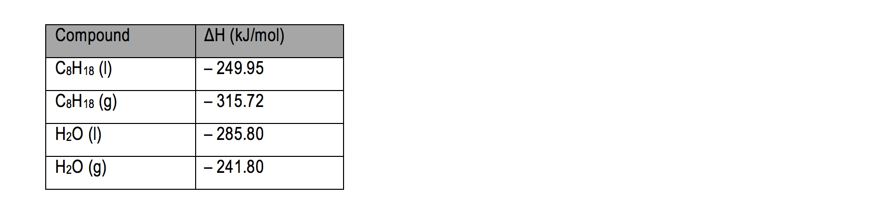# Problem: If the combustion of liquid octane, C 8H18, has a heat of reaction that is equal to –10.94 kJ, calculate the standard heat of formation of CO2.

###### FREE Expert Solution
95% (104 ratings)
###### Problem Details

If the combustion of liquid octane, C 8H18, has a heat of reaction that is equal to –10.94 kJ, calculate the standard heat of formation of CO2What scientific concept do you need to know in order to solve this problem?

Our tutors have indicated that to solve this problem you will need to apply the Enthalpy of Formation concept. You can view video lessons to learn Enthalpy of Formation. Or if you need more Enthalpy of Formation practice, you can also practice Enthalpy of Formation practice problems.

What is the difficulty of this problem?

Our tutors rated the difficulty ofIf the combustion of liquid octane, C 8H18, has a heat of re...as high difficulty.

How long does this problem take to solve?

Our expert Chemistry tutor, Dasha took 10 minutes and 11 seconds to solve this problem. You can follow their steps in the video explanation above.

What professor is this problem relevant for?

Based on our data, we think this problem is relevant for Professor Davis' class at OLE MISS.